# Tutorial¶

This tutorial assumes knowedge of the Python programming language, as well as familiarity with the third party modules Numpy and Matplotlib. It also assumes knowledge of advanced calculus, Einstein notation, weak formulations, and the Finite Element Method.

We will introduce fundamental Nutils concepts based on the 1D homogeneous Laplace problem,

$u''(x) = 0$

with boundary conditions $$u(0) = 0$$ and $$u'(1) = 1$$. Even though the solution is trivially found to be $$u(x) = x$$, the example serves to introduce many key concepts in the Nutils paradigm, concepts that can then be applied to solve a wide class of physics problems.

## A little bit of theory¶

Before turning to code we must first formally cast the problem into weak form.

Let $$Ω$$ be the unit line $$[0,1]$$ with boundaries $$Γ_\text{left}$$ and $$Γ_\text{right}$$, and let $$H_0(Ω)$$ be a suitable function space such that any $$u ∈ H_0(Ω)$$ satisfies $$u = 0$$ in $$Γ_\text{left}$$. The Laplace problem is solved uniquely by the element $$u ∈ H_0(Ω)$$ for which $$R(v, u) = 0$$ for all test functions $$v ∈ H_0(Ω)$$, with $$R$$ the bilinear functional

(1)$R(v, u) := ∫_Ω v_{,i} u_{,i} \ dx - ∫_{Γ_\text{right}} v \ dx.$

We next restrict ourselves to a finite dimensional subspace, to which end we adopt a set of Finite Element basis functions $$φ_n ∈ H_0(Ω)$$. In this space, the Finite Element solution is established by solving the linear system of equations $$R_n(\hat{u}) = 0$$, with residual vector $$R_n(\hat{u}) := R(φ_n, \hat{u})$$, and discrete solution

(2)$\hat{u}(x) = φ_n(x) \hat{u}_n.$

Note that discretization inevitably implies approximation, i.e. $$u ≠ \hat{u}$$ in general. In this case, however, we choose $$\{φ_n\}$$ to be the space of piecewise linears, which contains the exact solution. We therefore expect our Finite Element solution to be exact.

The computation can be set up in about 20 lines of Nutils code, including visualization. The entire script is presented below, in copy-pasteable form suitable for interactive exploration using for example ipython. In the sections that follow we will go over these lines ones by one and explain the relevant concepts involved.

>>> import nutils, numpy
>>> from matplotlib import pyplot as plt

>>> topo, geom = nutils.mesh.rectilinear([numpy.linspace(0, 1, 5)])

>>> ns = nutils.function.Namespace()
>>> ns.x = geom
>>> ns.basis = topo.basis('spline', degree=1)
>>> ns.u = 'basis_n ?lhs_n'

>>> sqr = topo.boundary['left'].integral('u^2 d:x' @ ns, degree=2)
>>> cons = nutils.solver.optimize('lhs', sqr, droptol=1e-15)
optimize > constrained 1/5 dofs
optimize > optimum value 0.00e+00

>>> res = topo.integral('basis_n,i u_,i d:x' @ ns, degree=0)
>>> res -= topo.boundary['right'].integral('basis_n d:x' @ ns, degree=0)
>>> lhs = nutils.solver.solve_linear('lhs', residual=res, constrain=cons)
solve > solving 4 dof system to machine precision using direct solver
solve > solver returned with residual 9e-16

>>> bezier = topo.sample('bezier', 32)
>>> nanjoin = lambda array, tri: numpy.insert(array.take(tri.flat, 0).astype(float), slice(tri.shape, tri.size, tri.shape), numpy.nan, axis=0)
>>> sampled_x = nanjoin(bezier.eval(ns.x), bezier.tri)
>>> def plot_line(func, **arguments):
...   plt.plot(sampled_x, nanjoin(bezier.eval(func, **arguments), bezier.tri))
...   plt.xlabel('x_0')
...   plt.xticks(numpy.linspace(0, 1, 5))

>>> plot_line(ns.u, lhs=lhs)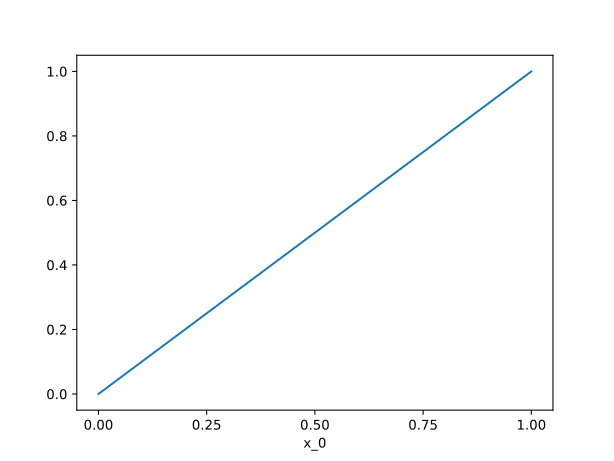You are encouraged to execute this code at least once before reading on, as the code snippets that follow may assume certain products to be present in the namespace. In particular the plot_line function is used heavily in the ensuing sections.

## Topology vs Geometry¶

Rather than having a single concept of what is typically referred to as the ‘mesh’, Nutils maintains a strict separation of topology and geometry. The nutils.topology.Topology represents a collection of elements and inter-element connectivity, along with recipes for creating bases. It has no (public) notion of position. The geometry takes the Topology and positions it in space. This separation makes it possible to define multiple geometries belonging to a single Topology, a feature that is useful for example in certain Lagrangian formulations.

While not having mesh objects, Nutils does have a nutils.mesh module, which hosts functions that return tuples of topology and geometry. Nutils provides two builtin mesh generators: nutils.mesh.rectilinear(), a generator for structured topologies (i.e. tensor products of one or more one-dimensional topologies), and nutils.mesh.unitsquare(), a unit square mesh generator with square or triangular elements or a mixture of both. The latter is mostly useful for testing. In addition to generators, Nutils also provides the nutils.mesh.gmsh() importer for gmsh-generated meshes.

The structured mesh generator takes as its first argument a list of element vertices per dimension. A one-dimensional topology with four elements of equal size between 0 and 1 is generated by

>>> nutils.mesh.rectilinear([[0, 0.25, 0.5, 0.75, 1.0]])
(StructuredTopology<4>, Array<1>)


Alternatively we could have used numpy.linspace() to generate a sequence of equidistant vertices, and unpack the resulting tuple:

>>> topo, geom = nutils.mesh.rectilinear([numpy.linspace(0, 1, 5)])


We will use this topology and geometry throughout the remainder of this tutorial.

Note that the argument is a list of length one: this outer sequence lists the dimensions, the inner the vertices per dimension. To generate a two-dimensional topology, simply add a second list of vertices to the outer list. For example, an equidistant topology with four by eight elements with a unit square geometry is generated by

>>> nutils.mesh.rectilinear([numpy.linspace(0, 1, 5), numpy.linspace(0, 1, 9)])
(StructuredTopology<4x8>, Array<2>)


Any topology defines a boundary via the Topology.boundary attribute. Optionally, a topology can offer subtopologies via the getitem operator. The rectilinear mesh generator automatically defines ‘left’ and ‘right’ boundary groups for the first dimension, making the left boundary accessible as:

>>> topo.boundary['left']
StructuredTopology<>


Optionally, a topology can be made periodic in one or more dimensions by passing a list of dimension indices to be periodic via the keyword argument periodic. For example, to make the second dimension of the above two-dimensional mesh periodic, add periodic=:

>>> nutils.mesh.rectilinear([numpy.linspace(0, 1, 5), numpy.linspace(0, 1, 9)], periodic=)
(StructuredTopology<4x8p>, Array<2>)


Note that in this case the boundary topology, though still available, is empty.

## Bases¶

In Nutils, a basis is a vector-valued function object that evaluates, in any given point $$ξ$$ on the topology, to the full array of basis function values $$φ_0(ξ), φ_1(ξ), \dots, φ_{n-1}(ξ)$$. It must be pointed out that Nutils will in practice operate only on the basis functions that are locally non-zero, a key optimization in Finite Element computations. But as a concept, it helps to think of a basis as evaluating always to the full array.

Several Topology objects support creating bases via the Topology.basis() method. A StructuredTopology, as generated by nutils.mesh.rectilinear(), can create a spline basis with arbitrary degree and arbitrary continuity. The following generates a degree one spline basis on our previously created unit line topology topo:

>>> basis = topo.basis('spline', degree=1)


The five basis functions are

>>> plot_line(basis)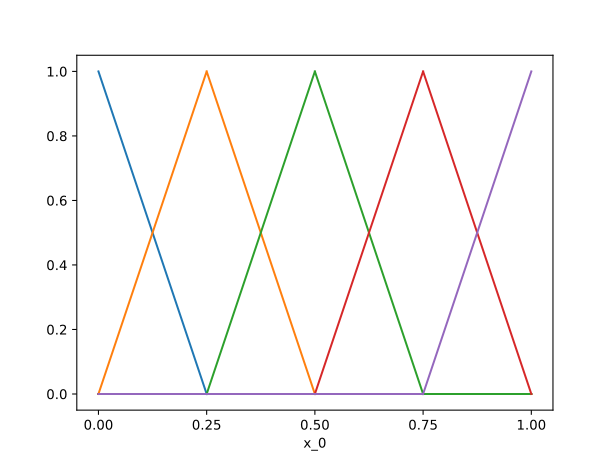We will use this basis throughout the following sections.

Change the degree argument to 2 for a quadratic spline basis:

>>> plot_line(topo.basis('spline', degree=2))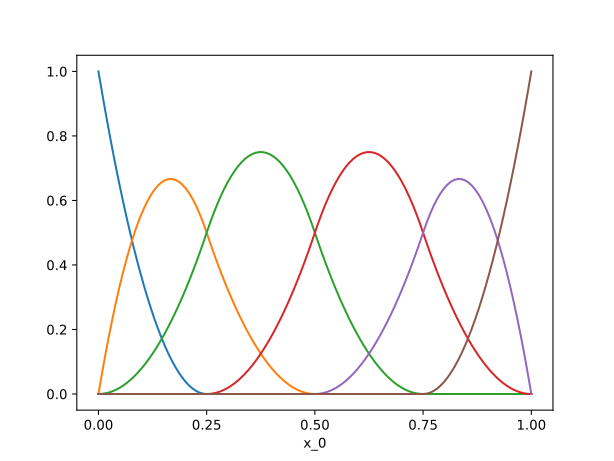By default the continuity of the spline functions at element edges is the degree minus one. To change this, pass the desired continuity via keyword argument continuity. For example, a quadratic spline basis with $$C^0$$ continuity is generated with

>>> plot_line(topo.basis('spline', degree=2, continuity=0))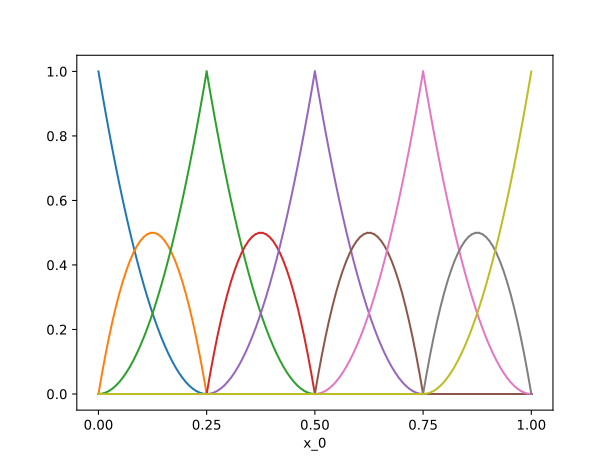$$C^0$$ continuous spline bases can also be generated by the 'std' basis:

>>> plot_line(topo.basis('std', degree=2))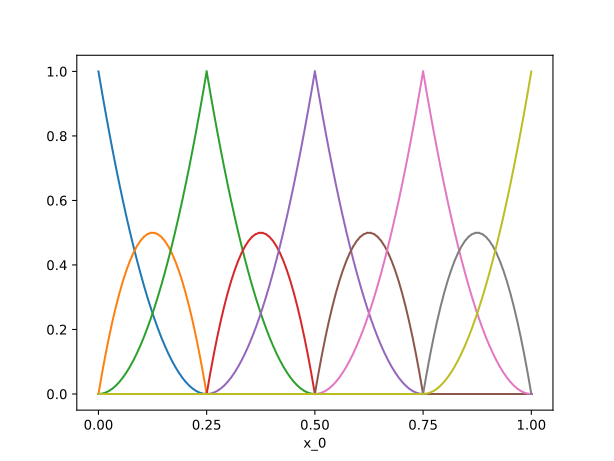The 'std' basis is supported by topologies with square and/or triangular elements without hanging nodes.

Discontinuous basis functions are generated using the 'discont' type, e.g.

>>> plot_line(topo.basis('discont', degree=2))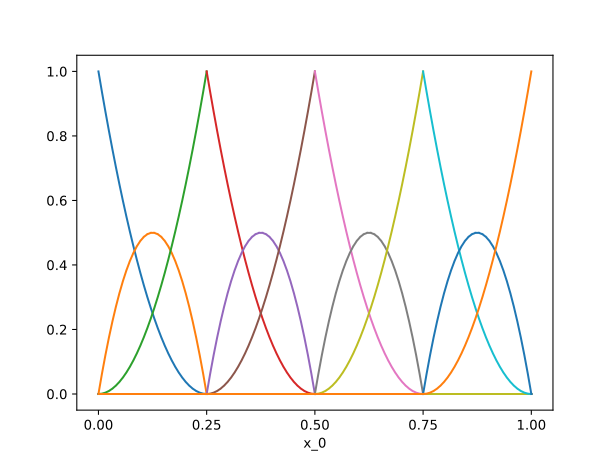## Functions¶

A function in Nutils is a mapping from a topology onto an n-dimensional array, and comes in the form of a functions: nutils.function.Array object. It is not to be confused with Python’s own function objects, which operate on the space of general Python objects. Two examples of Nutils functions have already made the scene: the geometry geom, as returned by nutils.mesh.rectilinear, and the bases generated by Topology.basis(). Though seemingly different, these two constructs are members of the same class and in fact fully interoperable.

The Array functions behave very much like numpy.ndarray objects: the functions have a shape, ndim and a dtype:

>>> geom.shape
(1,)
>>> basis.shape
(5,)
>>> geom.ndim
1
>>> geom.dtype
<class 'float'>


The functions support numpy-style indexing. For example, to get the first element of the geometry geom you can write geom and to select the first two basis functions you can write

>>> plot_line(basis[:2])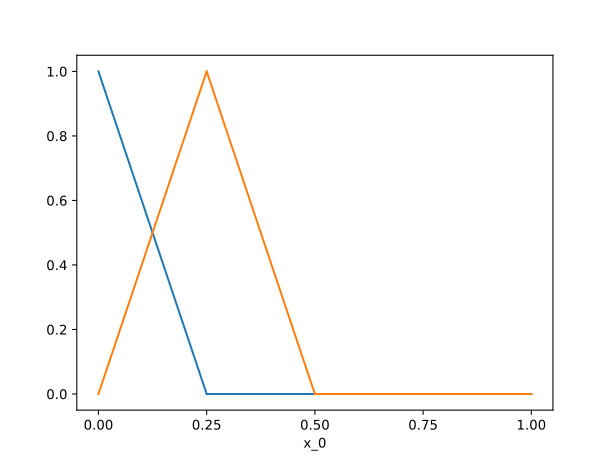The usual unary and binary operators are available:

>>> plot_line(geom*(1-geom)/2)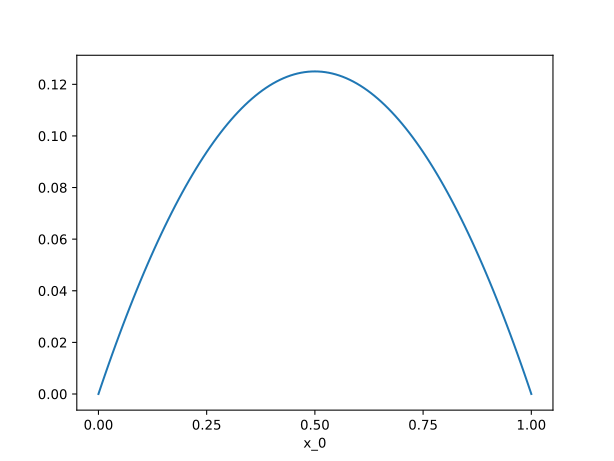Several trigonometric functions are defined in the nutils.function module. An example with a sine function:

>>> plot_line(nutils.function.sin(2*geom*numpy.pi))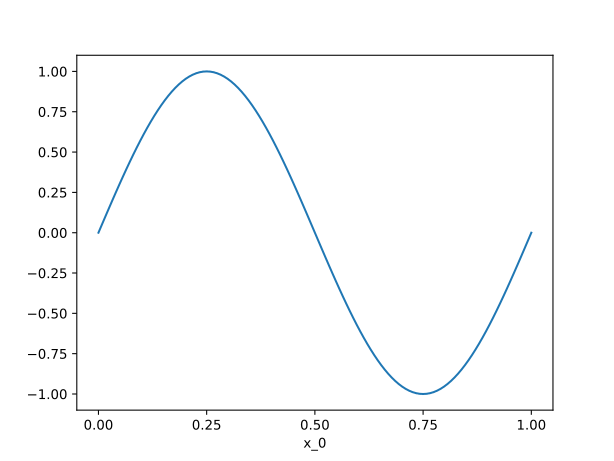The dot product is available via nutils.function.dot(). To contract the basis with an arbitrary coefficient vector:

>>> plot_line(nutils.function.dot(basis, [1,2,0,5,4]))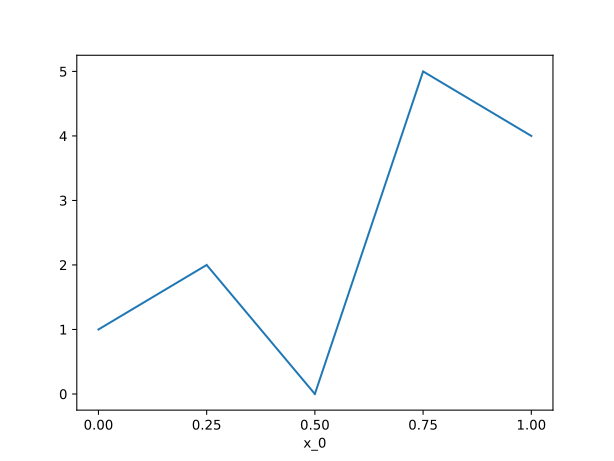Recalling the definition of our discrete solution (2), the above is precisely the way to evaluate the resulting function. What remains now is to establish the coefficients for which this function solves the Laplace problem.

## Namespace¶

Nutils functions behave entirely like Numpy arrays, and can be manipulated as such, using a combination of operators, object methods, and methods found in the nutils.function module. Though powerful, the resulting code is often lengthy, littered with colons and brackets, and hard to read. Namespaces provide an alternative, cleaner syntax for a prominent subset of array manipulations.

A nutils.function.Namespace is a collection of Array functions. An empty Namespace is created as follows:

>>> ns = nutils.function.Namespace()


New entries are added to a Namespace by assigning an Array to an attribute. For example, to assign the geometry geom to ns.x, simply type

>>> ns.x = geom


You can now use ns.x where you would use geom. Similarly, to assign a linear basis to ns.basis, type

>>> ns.basis = topo.basis('spline', degree=1)


You can also assign numbers and numpy.ndarray objects:

>>> ns.a = 1
>>> ns.b = 2
>>> ns.c = numpy.array([1,2])
>>> ns.d = numpy.array([[1,2],[3,4]])


### Expressions¶

In addition to inserting ready objects, a namespace’s real power lies in its ability to be assigned string expressions. These expressions may reference any Array function present in the Namespace, and must explicitly name all array dimensions, with the object of both aiding readibility and facilitating high order tensor manipulations. A short explanation of the syntax follows; see nutils.expression.parse() for the complete documentation.

A term is written by joining variables with spaces, optionally preceeded by a single number, e.g. 2 a b. A fraction is written as two terms joined by /, e.g. 2 a / 3 b, which is equivalent to (2 a) / (3 b). An addition or subtraction is written as two terms joined by + or -, respectively, e.g. 1 + a b - 2 b. Exponentation is written by two variables or numbers joined by ^, e.g. a^2. Several trigonometric functions are available, e.g. 0.5 sin(a).

Assigning an expression to the namespace is then done as follows.

>>> ns.e = '2 a / 3 b'
>>> ns.e = (2*ns.a) / (3*ns.b) # equivalent w/o expression


The resulting ns.e is an ordinary Array. Note that the variables used in the expression should exist in the namespace, not just as a local variable:

>>> localvar = 1
>>> ns.f = '2 localvar'
Traceback (most recent call last):
...
nutils.expression.ExpressionSyntaxError: Unknown variable: 'localvar'.
2 localvar
^^^^^^^^


When using arrays in an expression all axes of the arrays should be labelled with an index, e.g. 2 c_i and c_i d_jk. Repeated indices are summed, e.g. d_ii is the trace of d and d_ij c_j is the matrix-vector product of d and c. You can also insert a number, e.g. c_0 is the first element of c. All terms in an expression should have the same set of indices after summation, e.g. it is an error to write c_i + 1.

When assigning an expression with remaining indices to the namespace, the indices should be listed explicitly at the left hand side:

>>> ns.f_i = '2 c_i'
>>> ns.f = 2*ns.c # equivalent w/o expression


The order of the indices matter: the resulting Array will have its axes ordered by the listed indices. The following three statements are equivalent:

>>> ns.g_ijk = 'c_i d_jk'
>>> ns.g_kji = 'c_k d_ji'
>>> ns.g = ns.c[:,numpy.newaxis,numpy.newaxis]*ns.d[numpy.newaxis,:,:] # equivalent w/o expression


The gradient of a variable with respect to the default geometry — ns.x unless changed — is written by a comma followed by an index, e.g. the gradient of the basis is basis_n,i and the laplacian basis_n,ii. This works with expressions as well, e.g. (2 basis_n + basis_n^2)_,i is the gradient of 2 basis_n + basis_n^2.

The notation basis_n,i is actually shorthand for basis_n,x_i, in which form it is possible to take gradients to other geometries than the configured default.

### Manual evaluation¶

Sometimes it is useful to evaluate an expression to an Array without inserting the result in the namespace. For scalar or vector expressions, this can be done using the <expression> @ <namespace> notation. An example with a scalar expression:

>>> '2 a / 3 b' @ ns
Array<>
>>> (2*ns.a) / (3*ns.b) # equivalent w/o ... @ ns
Array<>


An example with a vector expression:

>>> '2 c_i' @ ns
Array<2>
>>> 2*ns.c # equivalent w/o ... @ ns
Array<2>


If an expression has more than one remaining index, the order of the indices must be specified explicitly. For this situation there is the <namespace>.eval_<indices>(<expression>) notation. An example:

>>> ns.eval_ijk('c_i d_jk')
Array<2,2,2>
>>> ns.c[:,numpy.newaxis,numpy.newaxis]*ns.d[numpy.newaxis,:,:] # equivalent w/o ns.eval_...(...)
Array<2,2,2>


### Arguments¶

A discrete model is often written in terms of an unknown, or a vector of unknowns. In Nutils this translates to a function argument, nutils.function.Argument. In an expression an Argument is denoted by a ? folowed by an identifier. For example, the discrete solution (2) can be written as

>>> ns.u = 'basis_n ?lhs_n'


with argument lhs the vector of unknowns $$\hat{u}_n$$. The shape of the argument lhs is resolved from the expression. In the above example, the argument lhs has the same shape as ns.basis.

## Integrals¶

A central operation in any Finite Element application is to integrate a function over a physical domain. In Nutils, integration starts with the topology, in particular the integral() method.

The integral method takes a Array function as first argument and the degree as keyword argument. The function should contain the Jacobian of the geometry against which the function should be integrated, using either nutils.function.J() or the d: operator in a namespace expression. For example, the following integrates 1 against the default geometry:

>>> I = topo.integral('1 d:x' @ ns, degree=0)
>>> I
Integral<>


The resulting nutils.sample.Integral object is a representation of the integral, as yet unevaluated. To compute the actual numbers, call the Integral.eval() method:

>>> I.eval()
1.0


Be careful with including the Jacobian in your integrands. The following two integrals are different:

>>> topo.integral('(1 + 1) d:x' @ ns, degree=0).eval()
2.0
>>> topo.integral('1 + 1 d:x' @ ns, degree=0).eval()
5.0


The Integral objects support additions and subtractions:

>>> J = topo.integral('x_0 d:x' @ ns, degree=1)
>>> (I+J).eval()
1.5


Recall that a topology boundary is also a Topology object, and hence it supports integration. For example, to integrate the geometry x over the entire boundary, write

>>> topo.boundary.integral('x_0 d:x' @ ns, degree=1).eval()
1.0


To limit the integral to the right boundary, write

>>> topo.boundary['right'].integral('x_0 d:x' @ ns, degree=1).eval()
1.0


Note that this boundary is simply a point and the integral a point evaluation.

Integrating and evaluating a 1D Array results in a 1D numpy.ndarray:

>>> topo.integral('basis_i d:x' @ ns, degree=1).eval()
array([0.125, 0.25 , 0.25 , 0.25 , 0.125])


Since the integrals of 2D Array functions are usually sparse, the Integral.eval() method does not return a dense numpy.ndarray, but a Nutils sparse matrix object: a subclass of nutils.matrix.Matrix. Nutils interfaces several linear solvers (more on this in Section Solvers below) but if you want to use a custom solver you can export the matrix to a dense, compressed sparse row or coordinate representation via the Matrix.export() method. An example:

>>> M = topo.integral(ns.eval_nm('basis_n,i basis_m,i d:x'), degree=1).eval()
>>> M
NumpyMatrix<5x5>
>>> M.export('dense')
array([[ 4., -4.,  0.,  0.,  0.],
[-4.,  8., -4.,  0.,  0.],
[ 0., -4.,  8., -4.,  0.],
[ 0.,  0., -4.,  8., -4.],
[ 0.,  0.,  0., -4.,  4.]])
>>> M.export('csr') # (data, column indices, row pointers)
(array([ 4., -4., -4.,  8., -4., -4.,  8., -4., -4.,  8., -4., -4.,  4.]),
array([0, 1, 0, 1, 2, 1, 2, 3, 2, 3, 4, 3, 4]),
array([ 0,  2,  5,  8, 11, 13]))
>>> M.export('coo') # (data, (row indices, column indices))
(array([ 4., -4., -4.,  8., -4., -4.,  8., -4., -4.,  8., -4., -4.,  4.]),
(array([0, 0, 1, 1, 1, 2, 2, 2, 3, 3, 3, 4, 4]),
array([0, 1, 0, 1, 2, 1, 2, 3, 2, 3, 4, 3, 4])))


## Solvers¶

Using topologies, bases and integrals, we now have the tools in place to start performing some actual functional-analytical operations. We start with what is perhaps the simplest of its kind, the least squares projection, demonstrating the different implementations now available to us and working our way up from there.

Taking the geometry component $$x_0$$ as an example, to project it onto the basis $$\{φ_n\}$$ means finding the coefficients $$\hat{u}_n$$ such that

$\left(∫_Ω φ_n φ_m \ dx\right) \hat u_m = ∫_Ω φ_n x_0 \ dx$

for all $$φ_n$$, or $$A_{nm} \hat{u}_m = f_n$$. This is implemented as follows:

>>> A = topo.integral(ns.eval_nm('basis_n basis_m d:x'), degree=2).eval()
>>> f = topo.integral('basis_n x_0 d:x' @ ns, degree=2).eval()
>>> A.solve(f)
solve > solving 5 dof system to machine precision using direct solver
solve > solver returned with residual 3e-17
array([0.  , 0.25, 0.5 , 0.75, 1.  ])


Alternatively, we can write this in the slightly more general form

$R_n := ∫_Ω φ_n (u - x_0) \ dx = 0.$
>>> res = topo.integral('basis_n (u - x_0) d:x' @ ns, degree=2)


Taking the derivative of $$R_n$$ to $$\hat{u}_m$$ gives the above matrix $$A_{nm}$$, and substituting for $$\hat{u}$$ the zero vector yields $$-f_n$$. Nutils can compute those derivatives for you, using the method Integral.derivative() to compute the derivative with respect to an Argument, returning a new Integral.

>>> A = res.derivative('lhs').eval()
>>> f = -res.eval(lhs=numpy.zeros(5))
>>> A.solve(f)
solve > solving 5 dof system to machine precision using direct solver
solve > solver returned with residual 3e-17
array([0.  , 0.25, 0.5 , 0.75, 1.  ])


The above three lines are so common that they are combined in the function nutils.solver.solve_linear():

>>> nutils.solver.solve_linear('lhs', res)
solve > solving 5 dof system to machine precision using direct solver
solve > solver returned with residual 3e-17
array([0.  , 0.25, 0.5 , 0.75, 1.  ])


We can take this formulation one step further. Minimizing

$S := ∫_Ω (u - x_0)^2 \ dx$

for $$\hat{u}$$ is equivalent to the above two variants. The derivative of $$S$$ to $$\hat{u}_n$$ gives $$2 R_n$$:

>>> sqr = topo.integral('(u - x_0)^2 d:x' @ ns, degree=2)
>>> nutils.solver.solve_linear('lhs', sqr.derivative('lhs'))
solve > solving 5 dof system to machine precision using direct solver
solve > solver returned with residual 6e-17
array([0.  , 0.25, 0.5 , 0.75, 1.  ])


The optimization problem can also be solved by the nutils.solver.optimize() function, which has the added benefit that $$S$$ may be nonlinear in $$\hat{u}$$ — a property not used here.

>>> nutils.solver.optimize('lhs', sqr)
optimize > solve > solving 5 dof system to machine precision using direct solver
optimize > solve > solver returned with residual 0e+00
optimize > optimum value 0.00e+00
array([0.  , 0.25, 0.5 , 0.75, 1.  ])


Nutils also supports solving a partial optimization problem. In the Laplace problem stated above, the Dirichlet boundary condition at $$Γ_\text{left}$$ minimizes the following functional:

>>> sqr = topo.boundary['left'].integral('(u - 0)^2 d:x' @ ns, degree=2)


By passing the droptol argument, nutils.solver.optimize() returns an array with nan (‘not a number’) for every entry for which the optimization problem is invariant, or to be precise, where the variation is below droptol:

>>> cons = nutils.solver.optimize('lhs', sqr, droptol=1e-15)
optimize > constrained 1/5 dofs
optimize > optimum value 0.00e+00
>>> cons
array([ 0., nan, nan, nan, nan])


Consider again the Laplace problem stated above. The residual (1) is implemented as

>>> res = topo.integral('basis_n,i u_,i d:x' @ ns, degree=0)
>>> res -= topo.boundary['right'].integral('basis_n d:x' @ ns, degree=0)


Since this problem is linear in argument lhs, we can use the nutils.solver.solve_linear() method to solve this problem. The constraints cons are passed via the keyword argument constrain:

>>> lhs = nutils.solver.solve_linear('lhs', res, constrain=cons)
solve > solving 4 dof system to machine precision using direct solver
solve > solver returned with residual 9e-16
>>> lhs
array([0.  , 0.25, 0.5 , 0.75, 1.  ])


For nonlinear residuals you can use nutils.solver.newton.

## Sampling¶

Having obtained the coefficient vector that solves the Laplace problem, we are now interested in visualizing the function it represents. Nutils does not provide its own post processing functionality, leaving that up to the preference of the user. It does, however, facilitate it, by allowing Array functions to be evaluated in samples. Bundling function values and a notion of connectivity, these form a bridge between Nutils’ world of functions and the discrete realms of matplotlib, VTK, etc.

The Topology.sample(method, ...) method generates a collection of points on the Topology, according to method. The 'bezier' method generates equidistant points per element, including the element vertices. The number of points per element per dimension is controlled by the second argument of Topology.sample(). An example:

>>> bezier = topo.sample('bezier', 2)


The resulting nutils.sample.Sample object can be used to evaluate Array functions via the Sample.eval(func) method. To evaluate the geometry ns.x write

>>> x = bezier.eval('x_0' @ ns)
>>> x
array([0.  , 0.25, 0.25, 0.5 , 0.5 , 0.75, 0.75, 1.  ])


The first axis of the returned numpy.ndarray represents the collection of points. To reorder this into a sequence of lines in 1D, a triangulation in 2D or in general a sequence of simplices, use the Sample.tri attribute:

>>> x.take(bezier.tri, 0)
array([[0.  , 0.25],
[0.25, 0.5 ],
[0.5 , 0.75],
[0.75, 1.  ]])


Now, the first axis represents the simplices and the second axis the vertices of the simplices.

If an Array function has arguments, those arguments must be specified by keyword arguments to Sample.eval(). For example, to evaluate ns.u with argument lhs replaced by solution vector lhs, obtained using nutils.solver.solve_linear() above, write

>>> u = bezier.eval('u' @ ns, lhs=lhs)
>>> u
array([0.  , 0.25, 0.25, 0.5 , 0.5 , 0.75, 0.75, 1.  ])


We can now plot the sampled geometry x and solution u using matplotlib, plotting each line in Sample.tri with a different color:

>>> plt.plot(x.take(bezier.tri.T, 0), u.take(bezier.tri.T, 0))
[...]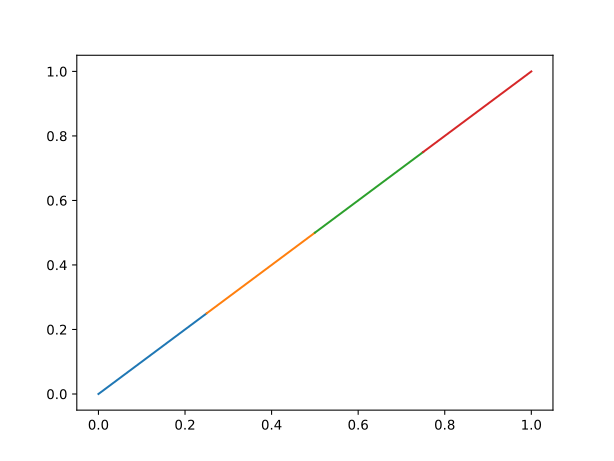Recall that we have imported matplotlib.pyplot as plt above. The plt.plot() function takes an array of x-values and and array of y-values, both with the first axis representing vertices and the second representing separate lines, hence the transpose of bezier.tri.

The plt.plot() function also supports plotting lines with discontinuities, which are represented by nan values. We can use this to plot the solution as a single, but possibly discontinuous line. The function numpy.insert() can be used to prepare a suitable array. An example:

>>> nanjoin = lambda array, tri: numpy.insert(array.take(tri.flat, 0).astype(float), slice(tri.shape, tri.size, tri.shape), numpy.nan, axis=0)
>>> nanjoin(x, bezier.tri)
array([0.  , 0.25,  nan, 0.25, 0.5 ,  nan, 0.5 , 0.75,  nan, 0.75, 1.  ])
>>> plt.plot(nanjoin(x, bezier.tri), nanjoin(u, bezier.tri))
[...]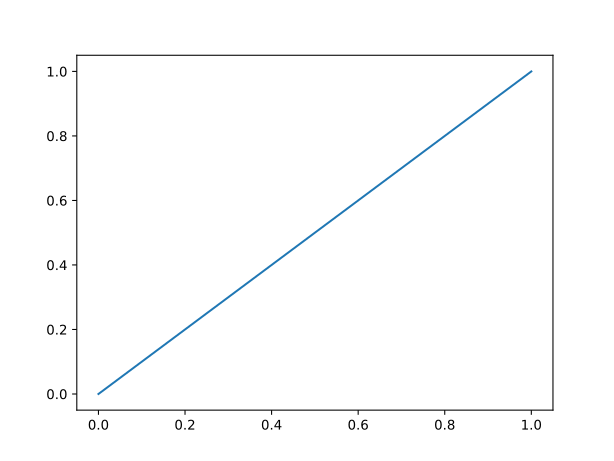Note the difference in colors between the last two plots.

## Two-dimensional Laplace problem¶

All of the above was written for a one-dimensional example. We now extend the Laplace problem to two dimensions and highlight the changes to the corresponding Nutils implementation. Let $$Ω$$ be a unit square with boundary $$Γ$$, on which the following boundary conditions apply:

\begin{align}\begin{aligned}u &= 0 && Γ_\text{left}\\u_{,i} n_i &= 0 && Γ_\text{bottom}\\u_{,i} n_i &= \cos(1) \cosh(x_1) && Γ_\text{right}\\ u &= \cosh(1) \sin(x_0) && Γ_\text{top}\end{aligned}\end{align}

The 2D homogeneous Laplace solution is the field $$u$$ for which $$R(v, u) = 0$$ for all v, where

(3)$R(v, u) := ∫_Ω v_{,i} u_{,i} \ dx - ∫_{Γ_\text{right}} v \cos(1) \cosh(x_1) \ dx.$

Adopting a Finite Element basis $$\{φ_n\}$$ we obtain the discrete solution $$\hat{u}(x) = φ_n(x) \hat{u}_n$$ and the system of equations $$R(φ_n, \hat{u}) = 0$$.

Following the same steps as in the 1D case, a unit square mesh with 10x10 elements is formed using nutils.mesh.rectilinear():

>>> nelems = 10
>>> topo, geom = nutils.mesh.rectilinear([numpy.linspace(0, 1, nelems+1), numpy.linspace(0, 1, nelems+1)])


Recall that nutils.mesh.rectilinear() takes a list of element vertices per dimension. Alternatively you can create a unit square mesh using nutils.mesh.unitsquare(), specifying the number of elements per dimension and the element type:

>>> topo, geom = nutils.mesh.unitsquare(nelems, 'square')


The above two statements generate exactly the same topology and geometry. Try replacing 'square' with 'triangle' or 'mixed' to generate a unit square mesh with triangular elements or a mixture of square and triangular elements, respectively.

We start with a clean namespace, assign the geometry to ns.x, create a linear basis and define the solution ns.u as the contraction of the basis with argument lhs.

>>> ns = nutils.function.Namespace()
>>> ns.x = geom
>>> ns.basis = topo.basis('std', degree=1)
>>> ns.u = 'basis_n ?lhs_n'


Note that the above statements are identical to those of the one-dimensional example.

The residual (3) is implemented as

>>> res = topo.integral('basis_n,i u_,i d:x' @ ns, degree=2)
>>> res -= topo.boundary['right'].integral('basis_n cos(1) cosh(x_1) d:x' @ ns, degree=2)


The Dirichlet boundary conditions are rewritten as a least squares problem and solved for lhs, yielding the constraints vector cons:

>>> sqr = topo.boundary['left'].integral('u^2 d:x' @ ns, degree=2)
>>> sqr += topo.boundary['top'].integral('(u - cosh(1) sin(x_0))^2 d:x' @ ns, degree=2)
>>> cons = nutils.solver.optimize('lhs', sqr, droptol=1e-15)
optimize > solve > solving 21 dof system to machine precision using direct solver
optimize > solve > solver returned with residual 3e-17
optimize > constrained 21/121 dofs
optimize > optimum value 4.32e-10


To solve the problem res=0 for lhs subject to lhs=cons excluding the nan values, we can use nutils.solver.solve_linear():

>>> lhs = nutils.solver.solve_linear('lhs', res, constrain=cons)
solve > solving 100 dof system to machine precision using direct solver
solve > solver returned with residual 2e-15


Finally, we plot the solution. We create a Sample object from topo and evaluate the geometry and the solution:

>>> bezier = topo.sample('bezier', 9)
>>> x, u = bezier.eval(['x_i', 'u'] @ ns, lhs=lhs)


We use plt.tripcolor to plot the sampled x and u:

>>> plt.tripcolor(x[:,0], x[:,1], bezier.tri, u, shading='gouraud', rasterized=True)
<...>
>>> plt.colorbar()
<...>
>>> plt.gca().set_aspect('equal')
>>> plt.xlabel('x_0')
Text(...)
>>> plt.ylabel('x_1')
Text(...)This two-dimensional example is also available as script: laplace.py.